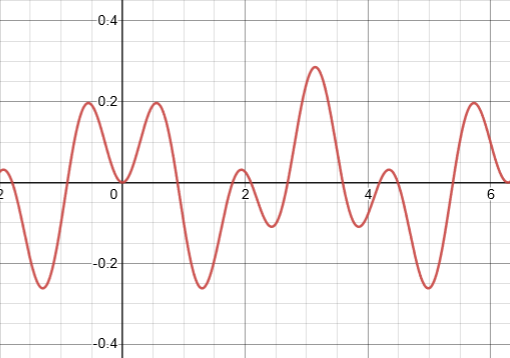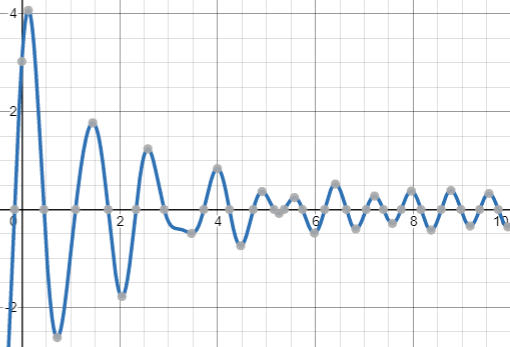## Sunday, November 14, 2021

### Problems 4: UNIZOR.COM - Physics4Teens - Waves - Mechanical Oscillations

Notes to a video lecture on http://www.unizor.com

Problems 4

Problem A

As presented in the lecture "Forced Oscillation 1", the general solution to a differential equation that describes forced oscillation looks like
x(t)=F0·cos(ω·t)/[m(ω0²−ω²)] +
+ D·cos(ω0·t−φ)

where
F0 is an amplitude of a periodic external force;
ω - angular speed of a periodic external force;
m - mass of an object on a spring;
k - elasticity of a spring;
ω0=√k/m is an inherent natural angular speed of oscillation of this particular object on this particular spring;
t is time;
D and φ parameters are determined by initial conditions for x(0) and x'(0).

Consider forced oscillations used as an example in that lecture.
A picture below represents forced oscillations of a system with its own natural angular speed ω0=2(rad/sec) with periodic force applied to it that has its angular speed ω=5(rad/sec).
Initial displacement and speed are at zero.Are the forced oscillations periodic?
If yes, what is the period of oscillations?

Solution

The result of a periodic force acting on periodic movement of the system is a periodic movement of this system but with a longer period.
If a period of a force is T1 and the own natural period of a system T2, then smallest number T that is a multiple of both T1 and T2 (if it exists!) will be a period of system with periodic external force acting on it.

Such a period will exist, if T1/T2 is a rational number.
Indeed, if T1/T2=m/n, then T=T1·n=T2·m will be divisible by T1, getting integer n as a result, and by T2, getting integer m as a result.

The period of an external force with angular speed ω=5(rad/sec) is
T1 = 2π/5(sec)
The natural period of a system with its own natural angular speed ωh=2(rad/sec) is
T2 = 2π/2 = π(sec)
In this case T=2π(sec) would be a smallest time interval that contains integer number of both periods T1 (T/T1=2) and T2 (T/T2=5).

The system will be in a periodic motion with a period T=2π(sec).

Problem B

Let's solve a problem that combines free oscillations, viscosity and external periodic force acting on an oscillating object.
Making all the reasonable assumptions, derive the equation of motion of such oscillations.

Solution

Let's assume that oscillations and all the forces are acting along the X-axis, so we have to derive a position of an object relative to the equilibrium point, which is at the origin of X-coordinate, as a function of time t.

If the position of an object relative to an equilibrium point is x(t), we assume the following:
(a) a spring acts on it, according to the Hooke's Law, with a force Fs(t)=−κ·x(t), where κ is a spring elasticity
(b) viscosity force is proportional to the speed x'(t) of an object and equals to Fv(t)=−c·x'(t), where c is viscosity damping coefficient
(wherever necessary, we will assume that viscosity c is sufficiently small in order to observe some oscillations)
(c) an external force is periodic and equals to Fx(t)=F0·cos(ω·t), where F0 is a constant amplitude and ω represents an angular speed of oscillations of an external force.

Now, according to the Newton's Second Law, the combination of all these forces should be equal to mass m of an oscillating object times its acceleration x"(t):
m·x"(t) = −κ·x(t) − c·x'(t) +
+ F0·cos(ω·t)

It can be transformed into more traditional format
x"(t)+(c/m)·x'(t)+(κ/m)·x(t) =
= (F0/m)·cos(ω·t)

This differential equation is not homogeneous because the right side contains some function that is not linearly dependent on x(t).
If two solutions of the above non-homogeneous differential equation are known, their difference is, obviously, a solution to a corresponding homogeneous equation
xh"(t) + (c/m)·xh'(t) +
+ (κ/m)·xh(t) = 0

Therefore, to find all solutions to a non-homogeneous differential equation, it is sufficient to find one particular solution xp(t) and add to it a general solution to a corresponding homogeneous equation xh(t).

Let's start by finding a general solution to the corresponding homogeneous differential equation
xh"(t) + (c/m)·xh'(t) +
+ (κ/m)·xh(t) = 0

It's a linear differential equation of the second order, so its general solution is any linear combination of any two linearly independent particular solutions.

Let's look for solutions to this differential equation among functions that look like this:
xh(t) = eγt
to find a pair of γ's (may be complex) that result in eγt to be a solution of our homogeneous differential equation.

Using this approach, we obtain
xh'(t) = γ·eγt
xh"(t) = γ²·eγt
Substituting this into our homogeneous equation:
γ²·eγt+(c/m)·γ·eγt+(κ/m)·eγt=0

Since eγt is not zero, we have a quadratic equation for γ
γ² + (c/m)·γ + (κ/m) = 0

Its discriminant is
Δ = (c/m)²−4κ/m
For sufficiently small (by assumption) viscosity c this discriminant will be negative.
So, our quadratic equation for γ will have two complex solutions:
γ1,2 = −c/(2m)±√(c/(2m))²−κ/m

Let
ωh² = −(c/(2m))² + k/m
Then
γ1,2 = −c/(2m)±√−ωh² =
= −c/(2m) ± ωh·i

where i is an imaginary unit, i²=−1

From this, the solution to our homogeneous differential equation, that does not take into account external periodic force, is
xh1,2(t) = eγ1,2·t =
= e
[−c/(2m)±ωh·i]·t =
= e−c·t/(2m)·e±ωh·i·t

The first member of this product e−c·t/(2m) is an exponentially diminishing amplitude of oscillation (because of viscosity).
The second one, according to Euler's formula, is
e±ωh·i·t = cos(ωh·t) ± i·sin(ωh·t)

If function z(t)=p(t)+i·q(t) with complex values satisfies some linear differential equation with real coefficients, its real and imaginary components must satisfy this equation independently.

Therefore,
xh1(t) = e−c·t/(2m)·cos(ωh·t)
and
xh2(t) = e−c·t/(2m)·sin(ωh·t)
are two independent solutions and the general solution to our linear homogeneous equation of the second order is any linear combination of these two solutions:
xh(t) = e−c·t/(2m)·
·
[A·cos(ωh·t)+B·sin(ωh·t)]
where A and B are any real numbers.

Using simple trigonometric identity
cos(α−β) =
= cos(α)·cos(β) + sin(α)·sin(β)

the above expression can be transformed into
xh(t) = R·e−c·t/(2m)·cos(ωh·t−φ)
where R = √A²+B² and
cos(φ)=A/R, sin(φ)=B/R.
Parameters R and φ are defined by initial conditions - initial displacement of an object from the equilibrium xh(0) and initial speed xh'(0).

Let's check that the above function is indeed a solution to our homogeneous differential equation
xh"(t) + (c/m)·xh'(t) +
+ (κ/m)·xh(t) = 0

Calculate all the components:
xh(t) = R·e−c·t/(2m)·cos(ωh·t−φ)

xh'(t) =
= −
[R·c/(2m)]·e−c·t/(2m)·
·cos(ωh·t−φ) −
[R·ωh]·e−c·t/(2m)·
·sin(ωh·t−φ)

xh"(t) =
=
[R·(c/(2m))²]·e−c·t/(2m)·
·cos(ωh·t−φ) +
+
[R·ωh·c/(2m)]·e−c·t/(2m)·
·sin(ωh·t−φ) +
+
[R·ωh·c/(2m)]·e−c·t/(2m)·
·sin(ωh·t−φ) −
[R·ωh²]·e−c·t/(2m)·
· cos(ωh·t−φ) =
= R·e−c·t/(2m)·
·
{[(c/(2m))²−ωh²]·cos(ωh·t−φ) +
+
[(c/m)·ωh]·sin(ωh·t−φ)}

(c/m)·xh'(t) =
= −
[R·c²/(2m²)]·e−c·t/(2m)·
·cos(ωh·t−φ) −
[R·ωh·c/m]·e−c·t/(2m)·
·sin(ωh·t−φ)

Since
κ/m = ωh² + (c/(2m))²
we get the following:
(κ/m)·xh(t) =
=
[ωh²+(c/(2m))²]·xh(t) =
=
[ωh²+(c/(2m))²]·R·e−c·t/(2m)·
·cos(ωh·t−φ)

Therefore,
xh"(t) + (c/m)·xh'(t) +
+ (κ/m)·xh(t) =
= R·e−c·t/(2m)·cos(ωh·t−φ)·X +
+ R·e−c·t/(2m)·sin(ωh·t−φ)·Y

where
X = (c/(2m))² − ωh² −
− c²/(2m²) + ωh²+(c/(2m))² =
= c²/(4m²) − ωh² −
− c²/(2m²) + ωh² + c²/(4m²) = 0

Y = (c/m)·ωh − ωh·c/m = 0

That proves that the general solution to our homogeneous differential equation of the second order is the function
xh(t) = R·e−c·t/(2m)·cos(ωh·t−φ)
where R is an amplitude and φ is a phase shift of oscillations, both determined by initial conditions xh(0) and xh'(0).

Now, to find all solutions to our original non-homogeneous differential equation
x"(t)+(c/m)·x'(t)+(κ/m)·x(t) =
= (F0/m)·cos(ω·t)

it is sufficient to find one particular solution xp(t) and add to it a general solution to a corresponding homogeneous equation xh(t) that we have found above.

Considering the trigonometric character of the right side of our original equation, let's try to find a particular solution in a form
xp(t) = A·cos(ω·t) + B·sin(ω·t)
where A and B are some constants.

Then
x'p(t) =
= −A·ω·sin(ω·t)+B·ω·cos(ω·t)

x"p(t) =
= −A·ω²·cos(ω·t)−B·ω²·sin(ω·t)

Substitute these functions into our original differential equation, getting −A·ω²·cos(ω·t)−B·ω²·sin(ω·t)−
−(c/m)·A·ω·sin(ω·t)+
+(c/m)·B·ω·cos(ω·t)+
+(κ/m)·A·cos(ω·t)+
+(κ/m)·B·sin(ω·t) =
= (F0/m)·cos(ω·t)

In order for this to be true for any time moment t, the corresponding coefficients at cos(.) and sin(.) functions on the left and right side of an equation must be equal.

The coefficient at cos(.) on the left side is supposed to be equal to (F0/m):
−A·ω²+(c/m)·B·ω+(κ/m)·A =
= (F0/m)

There is no sin(.) on the right side, so the coefficient at sin(.) on the left side is supposed to be equal to zero:
−B·ω²−(c/m)·A·ω+(κ/m)·B = 0

So, we have two linear equations with two variables A and B, which can easily be solved
−A·ω²+(c/m)·B·ω+(κ/m)·A =
= (F0/m)

−B·ω²−(c/m)·A·ω+(κ/m)·B =
= 0

Transform into canonical form
((k/m)−ω²)·A+(c/m)·ω·B =
= (F0/m)

−(c/m)·ω·A+((κ/m)−ω²)·B =
= 0

Solutions are:
A = (F0/m)·(k/m−ω²)/det
B = (F0/m)·(c/m)/det
where determinant
det = ((k/m)−ω²)² + (c/m)²·ω²

Now the general solution x(t) to our original non-homogeneous differential equation is a combination of the above partial solution
xp(t)=A·cos(ω·t)+B·sin(ω·t)
(where constants A and B are defined above)
and the general solution to a corresponding homogeneous solution
xh(t) = R·e−c·t/(2m)·cos(ωh·t−φ)

That is,
x(t) = xp(t) + xh(t) =
= A·cos(ω·t) + B·sin(ω·t) +
+ R·e−c·t/(2m)·cos(ωh·t−φ)

What's interesting about this analytical expression of such a complex oscillations is the fact that a component related to damped oscillations with the system's natural angular speed ωh is gradually decreasing in amplitude as time passes, because viscosity exponentially diminishes its amplitude R·e−c·t/(2m) to zero.
That leaves the oscillations with major angular speed that depends only on the driving angular speed ω of an external force.

The following graph renders the gradual transformation of damped oscillations under an external periodic force into oscillations mostly dependent on the angular speed of that external force and less and less dependent on the natural properties of a spring and environment.The parameters chosen for this graph are:
A = 0.2
B = 0.3
ω =8
R = 4
c = 1
m = 1
ωh=5
φ = π/4